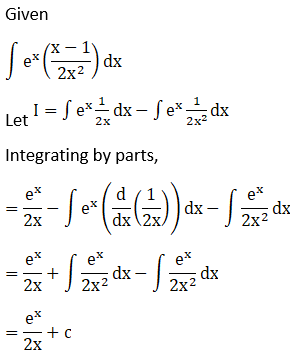# RD Sharma Solutions For Class 12 Maths Exercise 19.26 Chapter 19 Indefinite Integrals

RD Sharma Solutions for Class 12 Maths Exercise 19.26 Chapter 19 Indefinite Integrals is provided here. RD Sharma Solutions are developed by our expert tutors aiming to help students attain the correct methods of solving problems. In order to strengthen problem-solving abilities, make use of

RD Sharma Solutions, which are provided here, to achieve your goals. Students can download the PDF of RD Sharma Solutions for Class 12 Maths Chapter 19 Indefinite Integrals Exercise 19.26, from the links provided below. Here students will learn about evaluating the integral of the form

$$\begin{array}{l}\int e^{x}\left \{ f(x) +f^{‘}x \right \}\ dx\end{array}$$
by using integration by parts method.

## Download the PDF of RD Sharma Solutions For Class 12 Chapter 19 – Indefinite Integrals Exercise 19.26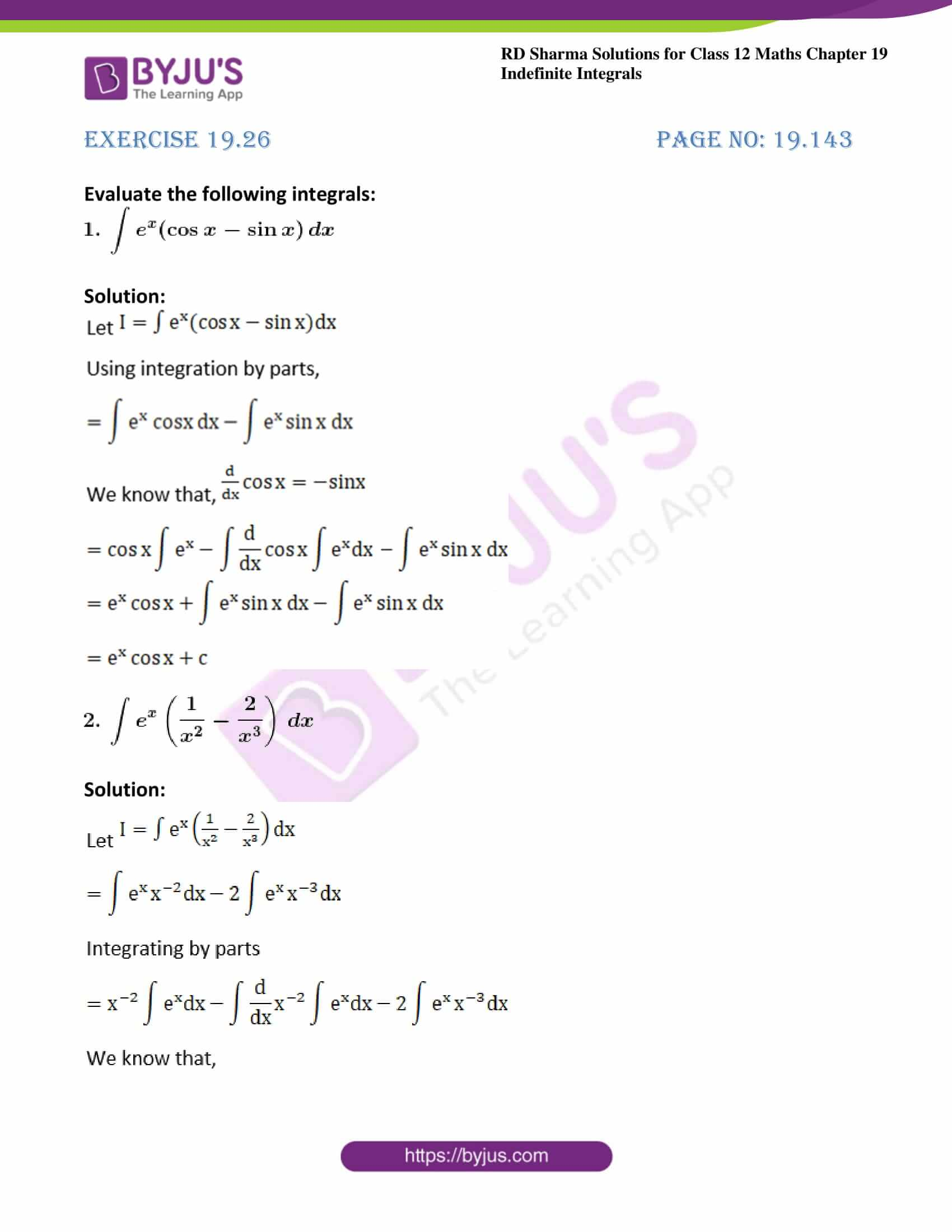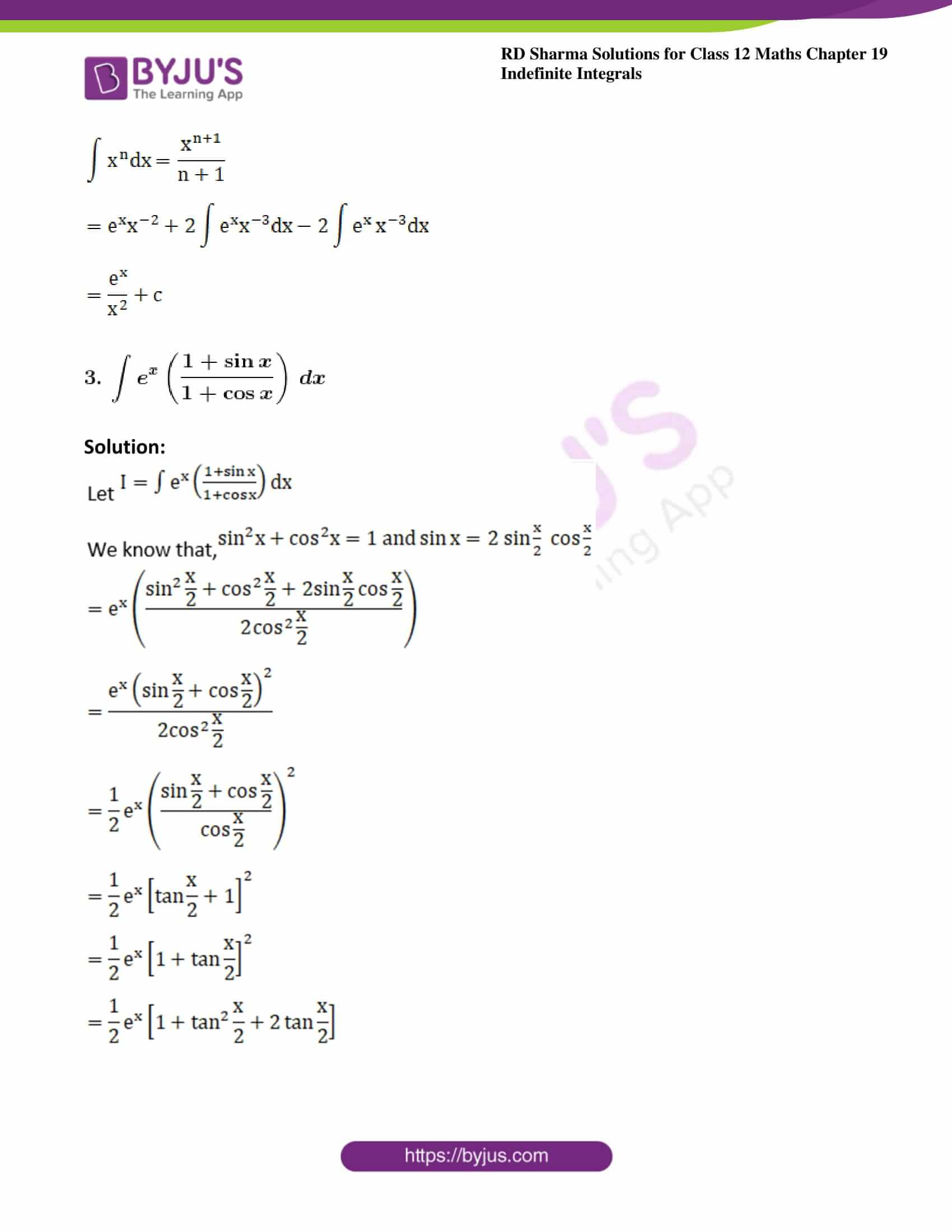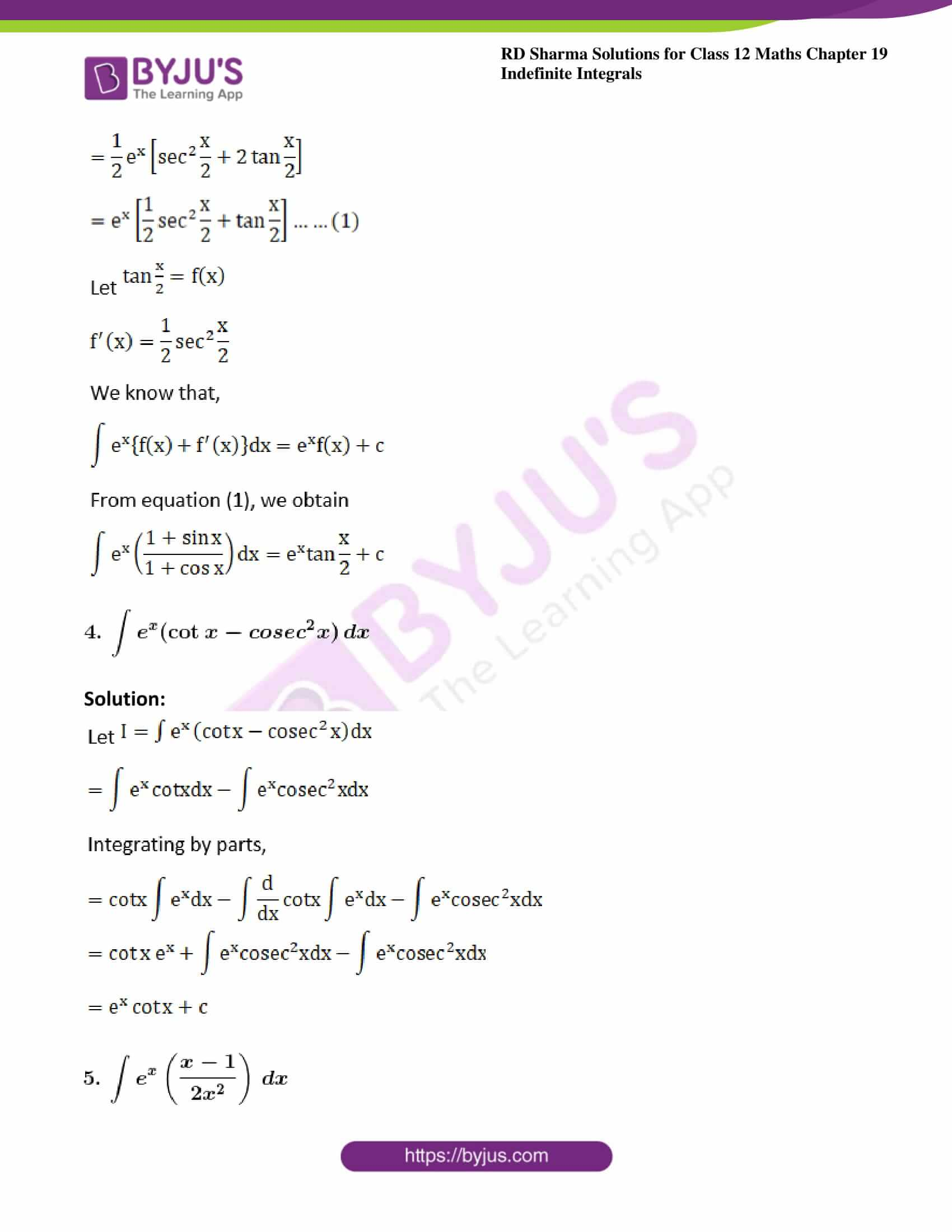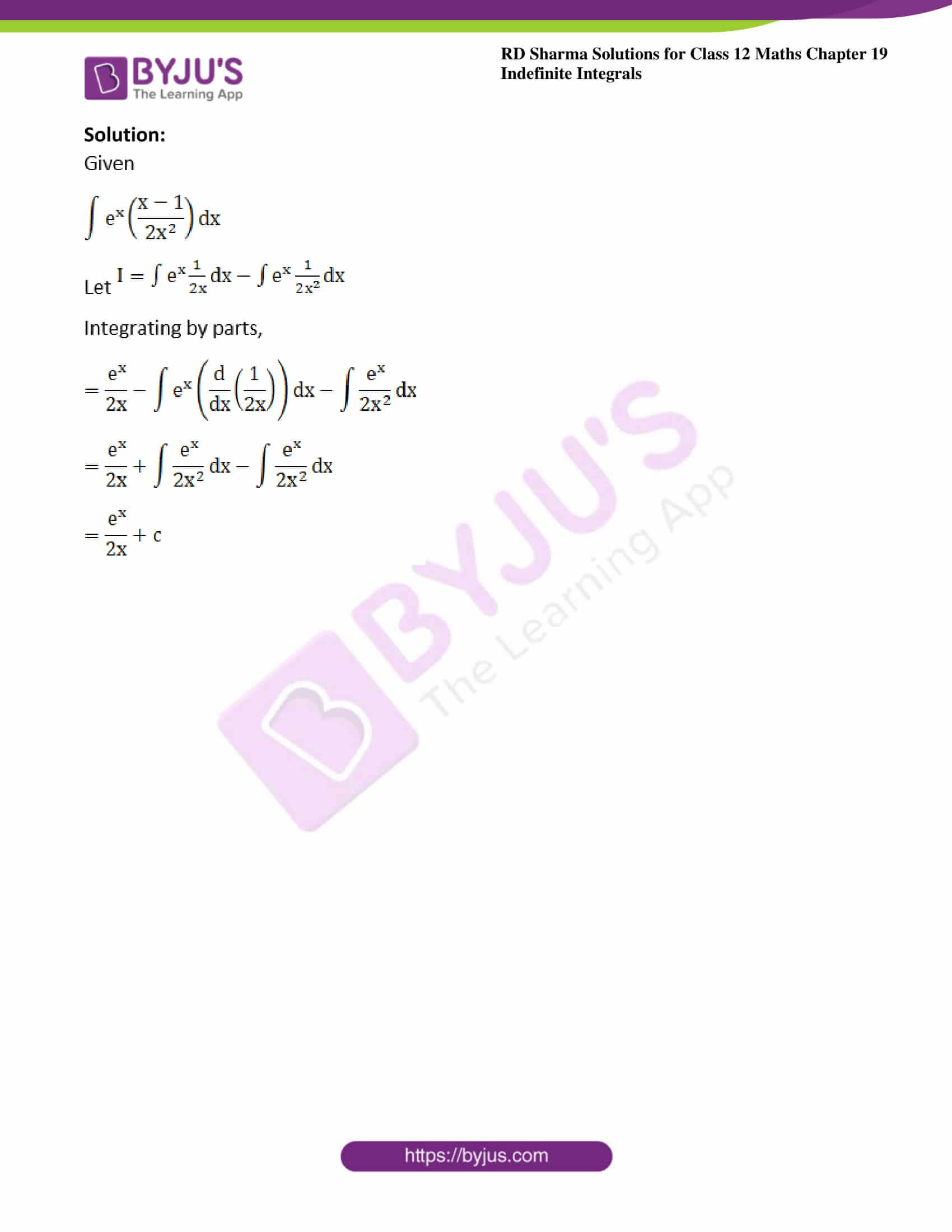### Access other exercises of RD Sharma Solutions For Class 12 Chapter 19 – Indefinite Integrals

Exercise 19.1 Solutions

Exercise 19.2 Solutions

Exercise 19.3 Solutions

Exercise 19.4 Solutions

Exercise 19.5 Solutions

Exercise 19.6 Solutions

Exercise 19.7 Solutions

Exercise 19.8 Solutions

Exercise 19.9 Solutions

Exercise 19.10 Solutions

Exercise 19.11 Solutions

Exercise 19.12 Solutions

Exercise 19.13 Solutions

Exercise 19.14 Solutions

Exercise 19.15 Solutions

Exercise 19.16 Solutions

Exercise 19.17 Solutions

Exercise 19.18 Solutions

Exercise 19.19 Solutions

Exercise 19.20 Solutions

Exercise 19.21 Solutions

Exercise 19.22 Solutions

Exercise 19.23 Solutions

Exercise 19.24 Solutions

Exercise 19.25 Solutions

Exercise 19.27 Solutions

Exercise 19.28 Solutions

Exercise 19.29 Solutions

Exercise 19.30 Solutions

Exercise 19.31 Solutions

Exercise 19.32 Solutions

### Exercise 19.26 Page No: 19.143

Evaluate the following integrals: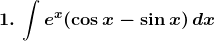Solution: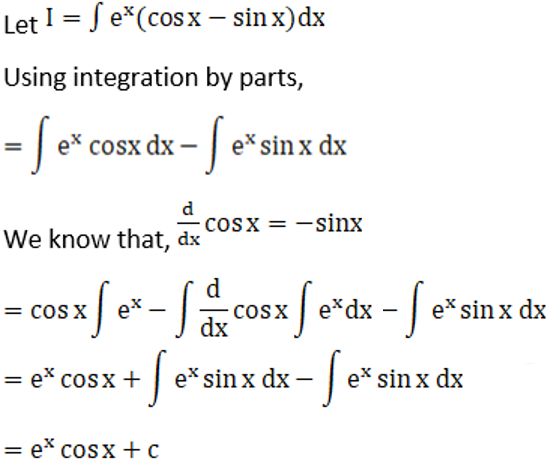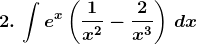Solution: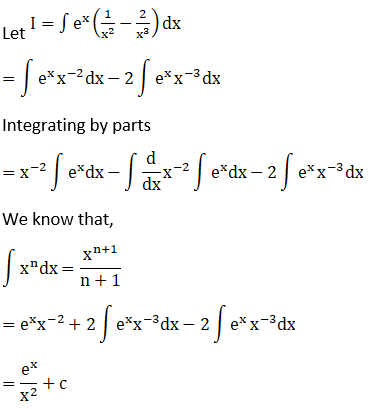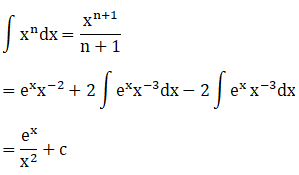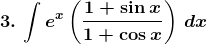Solution: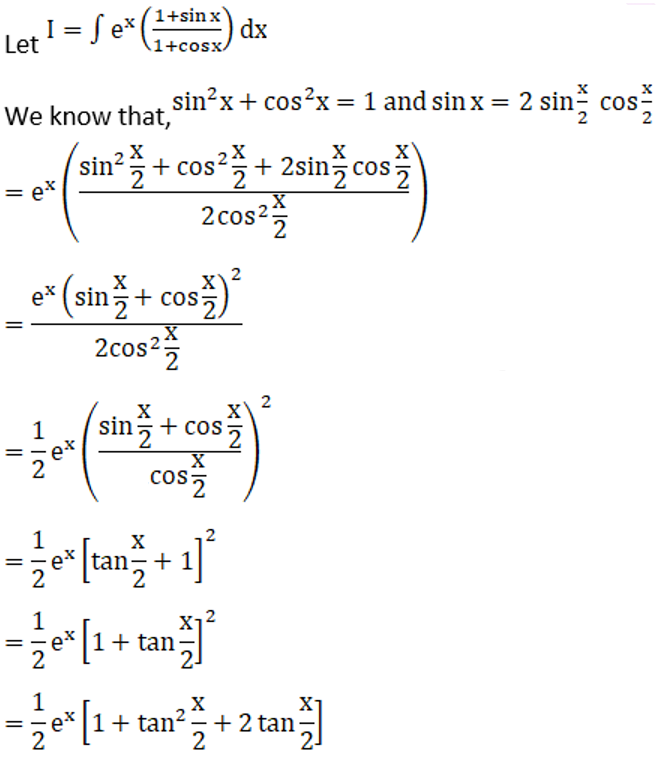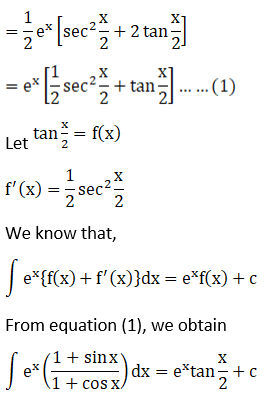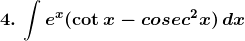Solution: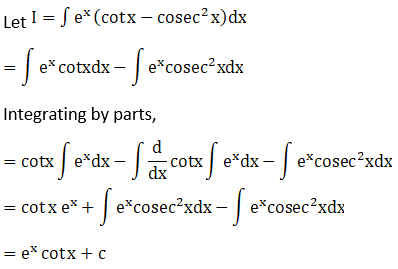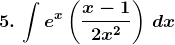Solution: